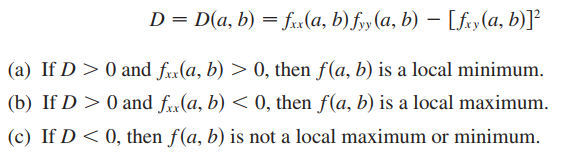Need Help?

Subscribe to Calculus C

###### \${selected_topic_name}
• Notes
• Critical points: هى النقاط التى تكون عندها مشتقة الدالة تساوى صفر او غير موجودة .
• A function of two variables has a local maximum at
(a, b) if f (x, y) =< f (a, b) when (x, y) is near (a, b)
• A function of two variables has a local minimum at
(a, b) if f (x, y) >= f (a, b) when (x, y) is near (a, b)
• Second Derivatives Test:• To find the absolute maximum and minimum values of a continuous function f on a closed, bounded set D:
1. Find the values of F at the critical points of F in D.
2. Find the extreme values of  F on the boundary of D.
3. The largest of the values from steps 1 and 2 is the absolute maximum value; the smallest of these values is the absolute minimum value